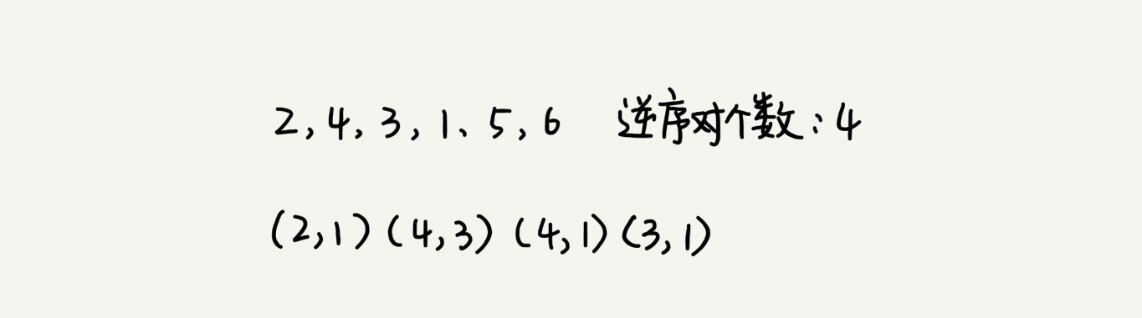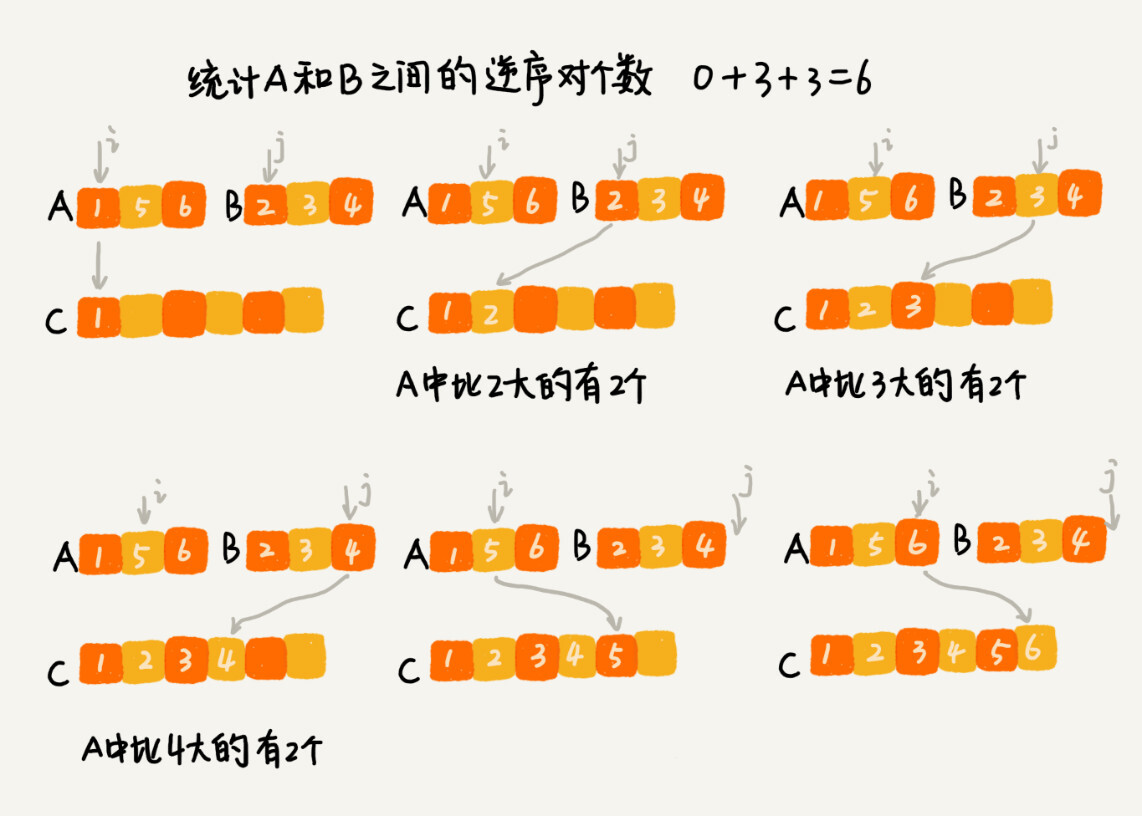MapReduce 是 Google 大数据处理的三驾马车之一，另外两个是 GFS 和 Bigtable。它在倒排索引、PageRank 计算、网页分析等搜索引擎相关的技术中都有大量的应用。

## 分治算法应用举例分析```private int num = 0; // 全局变量或者成员变量

public int count(int[] a, int n) {
num = 0;
mergeSortCounting(a, 0, n-1);
return num;
}

private void mergeSortCounting(int[] a, int p, int r) {
if (p >= r) return;
int q = (p+r)/2;
mergeSortCounting(a, p, q);
mergeSortCounting(a, q+1, r);
merge(a, p, q, r);
}

private void merge(int[] a, int p, int q, int r) {
int i = p, j = q+1, k = 0;
int[] tmp = new int[r-p+1];
while (i<=q && j<=r) {
if (a[i] <= a[j]) {
tmp[k++] = a[i++];
} else {
num += (q-i+1); // 统计p-q之间，比a[j]大的元素个数
tmp[k++] = a[j++];
}
}
while (i <= q) { // 处理剩下的
tmp[k++] = a[i++];
}
while (j <= r) { // 处理剩下的
tmp[k++] = a[j++];
}
for (i = 0; i <= r-p; ++i) { // 从tmp拷贝回a
a[p+i] = tmp[i];
}
}```